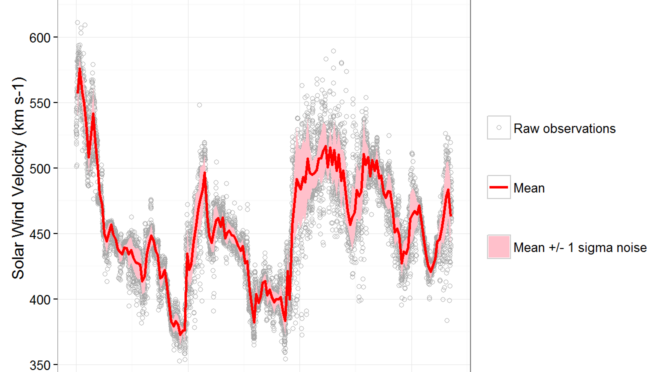# Solar wind observations by EPAM ACEThis is a part of study described in the dedicated post.

We have per minute solar wind observations (density, temperature and velocity) recorded by EPAM instrument of Advanced Composition Explorer (ACE) spacecraft. (see this link for data files)

This data from ACE can be used for two purposes. First, we can use this particular archive of observations (e.g. 2015 year) to fit the prediction model parameters. Second, we can use very recent measurements coming from ACE as predictors for forecasting the wind velocity for near future.

Having per minute measurements are too fine grained. Using solar wind observations in such form brings the noise to the machine learning algorithms which is bad.
I want to aggregate the data hourly. For each hour I want to get separate “trend” value and noise level for each of three data variables (density, temperature, velocity).

Thus we will get

• density_mean
• density_noise_sd
• temperature_mean
• temperature_noise_sd
• velocity_mean
• velocity_noise_sd

for every hour.

We will estimate trend by running median over the data. And in this case noise can be estimates by subtracting trend from original signal.
Then the data is aggregated in hourly manner.

Here I present the plots for the first week and for the first month of 2015 year.

It is worth to notice that temperature trend values go beyond zero sometimes. This implies that the data should be additionally coerced before usage in machine learning.

The resulting data sets are published here.

Complete R report is available here.